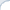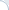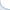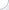## Unix pipes have small capacity

There is a catch in a previously described solution to wrapping NLP tools. Namely, a Unix pipe has a small buffer (I believe it is in the order of kilobytes). This is why you need to send input data in small chunks and read all the output after each chunk is processed. Otherwise, a deadlock can happen.

If you try to push a large chunk, a write call (that writes data to the output pipe) will be waiting till your application reads data from the other side of the output pipe. At the same time, your application will be trying to push input data through the input pipe. Because the pipe has a limited capacity, your application will exhaust this capacity and "freeze" in the write call. It will be waiting for the NLP tool to read the data from the other side of the input pipe. Yet, the NLP tool, in turn, will be waiting for your application!

As Di Wang pointed out, you can avoid this situation by using a temporary file and a pipe in a clever way. As in the naive and unsafe solution described in the beginning of the post, you will write the input data to a temporary file. Then, you will write the name of this temporary file to the input pipe. Because the name of the file is very small, you will not exceed the pipe capacity.

## Plumbing with named pipes: a simple approach to wrap standalone NLP tools

A modus operandi of many natural language processing (NLP) tools is as follows: start a tool, read raw data from the standard input, write processed data (e.g., POS tags) to the standard output and finish. That is, a lot of NLP tools come without any server mode. At the same time the cost of starting a process can be substantial. Thus, if you have to supply raw data in small chunks, the processing can be very slow.

What is the easiest way to fix this, if we do not care about multi-threading? Writing a fully-fledged network daemon is possible, but is rather hard. Some folk default to modifying the NLP tool so that it reads input from a file as the input appears. A modus operandi is, then, as follows. Sit and wait till the input file is created. When it happens, read raw data and output processed data to some other file. It is an easy solution: synchronization can be problematic though: We may end up with one process reading from the file while another one is writing to it.

A much better way is to use a named pipe! Unix named pipes are almost identical to regular temporary pipes that we use to direct an output of one process to an input of another process (using the symbol |). The only difference is that named pipes are permanent. From a perspective of a Unix process, a named pipe is just a regular pipe from which you can read data (or write data to). A benefit of using a pipe is that the operating system takes care of synchronization: one process can safely read from the pipe while another process is writing to it.

To begin the plumbing, we need to create two named pipes, one for input, another for output:
 mkfifo input_pipe mkfifo output_pipe

Then, we need to modify our NLP tool.

1) We make the tool process data in an infinite loop (rather than exiting after processing all the input). In the beginning of the loop, we open the the output pipe for writing (and we open the input pipe only once, when the tool starts). After all data is processed, we close the output pipe. Note that closing and re-opening the output pipe is important. Otherwise, a receiving process will not read the EOF marker and consequently, will wait for ever.
2) We replace all operators that read raw data from the standard input with operators that read data from the input named pipe.
3) We replace all operators that write processed data to the standard output with operators that write data to the output named pipe.

In C/C++, this is straightforward (in other languages this is not hard either). For instance, we replace

fgets(sentence, MAX_SENTENCE_SIZE, stdin)

with
fgets(sentence, max_sent_size + 1, senna_input)

That is, pretty much it. As a working example, I publish the modified main C-file of the SENNA parser version 3.0.

Happy plumbing and let your pipes be functioning properly!

PS1: There is one catch: in a previously described solution to wrapping NLP tools. Namely, a Unix pipe has a small buffer (I believe it is in the order of kilobytes). This is why you need to send input data in small chunks and read all the output after each chunk is processed. Otherwise, a deadlock can happen.

If you try to push a large chunk, a write call (that writes data to the output pipe) will be waiting till your application reads data from the other side of the output pipe. At the same time, your application will be trying to push input data through the input pipe. Because the pipe has a limited capacity, your application will exhaust this capacity and "freeze" in the write call. It will be waiting for the NLP tool to read the data from the other side of the input pipe. Yet, the NLP tool, in turn, will be waiting for your application!

As Di Wang pointed out, you can avoid this situation by using a temporary file and a pipe in a clever way. As in the naive and unsafe solution described in the beginning of the post, you will write the input data to a temporary file. Then, you will write the name of this temporary file to the input pipe. Because the name of the file is very small, you will not exceed the pipe capacity.

PS2: In principle, even a simpler approach might be possible. And this approach would not require modification of the NLP tool. We can create a wrapper utility that would simulate the following Unix command:
 printing_binary | ./nlp_tool_binary | recipient_binary
A trick is to implement this pipeline in such a way that printing_binary is the same as the recipient_binary. In C/C++ and Unix, it is possible, e.g., through a system call popen. One big issue here, though, is that we need to feed several input batches to nlp_tool_binary. However, the output from nlp_tool_binary can be a single stream of characters/bytes without separators that indicate batch boundaries.

Clearly, we need to pause after feeding every input batch until we retrieve all the respective output data. However, if we keep reading the output of nlp_tool_binary using a blocking read operation, we will eventually end up waiting forever. We can read using a non-blocking system call, but how long should we repeat this system call before giving up and declaring that nlp_tool_binary has processed all the data? It might be possible to use some knowledge about the output format of nlp_tool_binary, or simply stop trying after a certain time elapses. Yet, none of these solutions is sufficiently reliable or generic, so if anybody knows a better approach, please, let me know.

## A small clarification on the LSH post

This is a clarification to the previous post. Note that I am not claiming that the LSH analysis is wrong! I am saying that apparently the analysis uses a simplifying assumption. And this assumption was swept under the carpet for many years (which is quite surprising). See also a discussion in the blog of Daniel Lemire.

Yet, such approaches are quite common in CS, statistics, physics, etc... As Daniel Lemire pointed out, an analysis of regular hash tables is essentially based on a very similar assumption: that a hash function distributes elements more or less randomly. I believe that in practice this is true only to a certain a degree (and this highly depends on a hash function). Yet, we still use the analysis. So, it is probably not a big deal.

I have recently come across another (actually well-known paper), where they talk at length about the assumptions being reasonable, but make a simple math error (or at least me and my co-author think so). So, it is infinitely better to rely (on perhaps silent) assumptions, but to get the math right, then to substantiate the assumptions and totally screw the math.﻿

## Does Locality Sensitive Hashing (LSH) analysis have a fatal flaw?

Preamble: Hongya Wang and colleagues have written a thought-provoking paper where they argue that performance analysis of a popular method: a Locality Sensitive Hashing (LSH) has a fatal flaw . Here is my take on this: even though their logic seems to be correct, I do not fully agree with their conclusions. In what follows, I consider this issue in more detail. See also my note here and a discussion in the blog of Daniel Lemire.

A locality-sensitive hashing, commonly known as LSH, is a popular method to compute locality-sensitive, distance-aware, hash values. More specifically, given two objects $x$ and $y$, the probability that their locality-sensitive hash values $h(x)$ and $h(y)$ are the same, depends on the distance between $x$ and $y$. The smaller is the distance, the more likely $x$ and $y$ collide with respect to the hash function $h()$. An event of getting $x$ and $y$ such that $h(x)=h(y)$ is called a collision.

This kind of distance sensitivity is a super-useful property, which fuels many probabilistic algorithms including approximate counting, clustering, and searching. In particular, with a good choice of a locality-sensitive function, we can build a hash table where close objects tend to be in the same bucket (with a sufficiently high probability). Thus, elements stored in the same bucket are good candidates for placing in the same cluster. In addition, given a query object $q$, potential nearest neighbors (again with some probability) can be found in the bucket corresponding to the hash value $h(q)$. If a collision probability for a single hash function is low, we need to build several hash tables over the same set. If we miss the nearest neighbor in one hash table, we still have a chance to find it another one. The more hash tables we build, the higher is a probability of success.2 Clearly, we need a family of locality-sensitive hash functions, but how do we get one?

In one specific, but important, case, we deal with bit (i.e., binary) vectors where dissimilarity is computed using the Hamming distance (the distance between bit vectors $x$ and $y$ is equal to the number of non-matching bits). In this setup, we can "create" binary hash functions by simply taking the value of an i-th bit. One can see that this is a projection to the 1-dimensional sub-space. The number of such functions $h^i()$ is equal to the length of a bit vector. Formally, the hash function number $i$ is defined as: $h^i(x) = x_i$.

This function is locality sensitive: The larger is the number of matching bits between vectors, the higher is the probability that they have equal i-th bits. What is the exact probability of a collision in this case? Typically, it is claimed to be $\frac{n - d}{n}$, where $n$ is the length of vectors and $d$ is the Hamming distance. For example, if vectors differ only in one bit, there is apparently $n$ ways to randomly select a projection dimension and, consequently, $n$ ways to select a hash function. Because, vectors differ only in a single bit number, only one choice of the hash function produces non-matching values. With respect to the remaining hash function, both vectors will collide. Thus, the collision probability is $\frac{n-1}{n}$.

Here is a subtle issue. As noted by Hongya Wang et al. , we do not randomly select a hash function for a specific pair of objects. Rather, we select hash functions randomly in advance and only afterwards we randomly select objects. Thus, we have a slightly different selection model than the one used in the previous paragraph! Using simple math, one can verify that if the set of objects comprises all possible bit vectors (and these bit vectors are selected randomly and uniformly), the two selection models are equivalent.

However, in general, these selection models are not equivalent. Consider a data set of bit vectors, such that their n-th bit is always set to one. For the first $n-1$ bits, all combinations are equally likely. Note that we essentially consider an (n-1)-dimensional sub-space with equi-probable elements. In this sub-space, two selection models are equivalent. Thus, a probability of a collision is $\frac{n - 1 - d}{n-1}$.

Note that this is true only for the first $n-1$ hash functions. Yet, for the function $h^n()$ the probability of a collision is different, namely it is one! Why? Because, we have a biased data set, where the value of the last (n-th) bit in each vector is always equal to one. Hence, a projection to the n-th dimension always results in a collision (for any pair of objects).

Hongya Wang et al.  go even further and carry out simulations. These simulations support their theoretical observations: The probabilities obtained via two different selection models are not always the same. So, it looks like the analysis of the LSH methods does rely on the simplifying assumption: The probability of a collision can be computed assuming that a hash function is randomly selected while the objects are fixed. Yet, I think that this simplification does not make a big difference, and it is the paper by Hongya Wang and colleagues that makes me think so.

First, even though collision ratios obtained via simulations (see Table 1 and Table 2 in the paper ) are sometimes quite different from predicted theoretical values, most of the time they are close to the theoretical predictions (again, based on the assumption that a hash function is selected randomly while objects are fixed). Second, as shown in Theorem 1, the average collision ratio rarely diverges from the theoretical value, if the number of objects and hash functions is large (which is mostly true in practice). Finally, I do not think that data sets where a significant fraction of the objects collide with respect to a given locality-sensitive hash function are likely. Such "mass" collisions might be a concern in some adversarial scenario (e.g., in the case of a DOS attack), but I would not expect this to happen under normal circumstances.

One nice property of the LSH is that we can tune parameters of the method to achieve a certain level of recall. Furthermore, these parameters can be selected based on the distribution of data . Thus, it would be possible to retrieve a true nearest neighbor in, e.g., about 50%, of all searches (in the shortest possible time). What happens if we miss the true answer? It is desirable that we still get something close. Yet, it turns out, that we often get results that are far from the nearest neighbor. This observation stems primarily from my own experiments , but there other papers where authors came to similar conclusions .

Thus, it may not be always possible to rely solely on the LSH in the nearest neighbor search. Streaming first story detection is one example, where authors use a hybrid method, in which an LSH-based search is a first step . If the LSH can find a tweet that is reasonably close to the query, the tweet topic is considered to be repetitive (i.e., it is not new). However, if we fail to find a previously created close-topic tweet using the LSH, we cannot be sure that the close-topic tweet does not exist. Why? The authors surmise that this may happen when the answer is not very close to the query. However, it might also be because the LSH tend to return a distant tweet even if a close-topic tweet exists in the index. To eliminate such potential false negatives, the authors use a small inverted file, which is built over a set of recent tweets.

To summarize, LSH is a great practical method based on reasonable theoretical assumptions, whose performance was verified empirically. It is possible to tune the parameters so that a certain recall level is achieved. Yet, there is seemingly no control in regard to how far are the objects in a result set from true nearest neighbors, when these true neighbors elude the LSH search algorithm.

1) This is a bit simplified description. In the real LSH, a hash function is often built using several elementary and binary hash functions, each of which is locality sensitive. In the case of real-valued vectors, one common approach to obtain binary functions is through a random projection.

## Pages